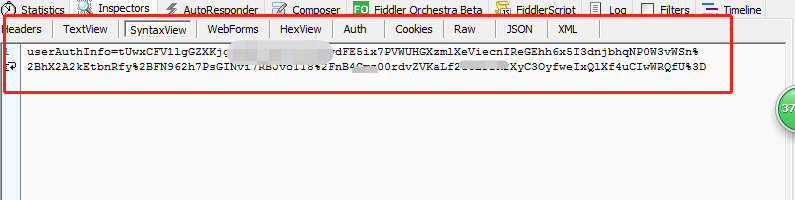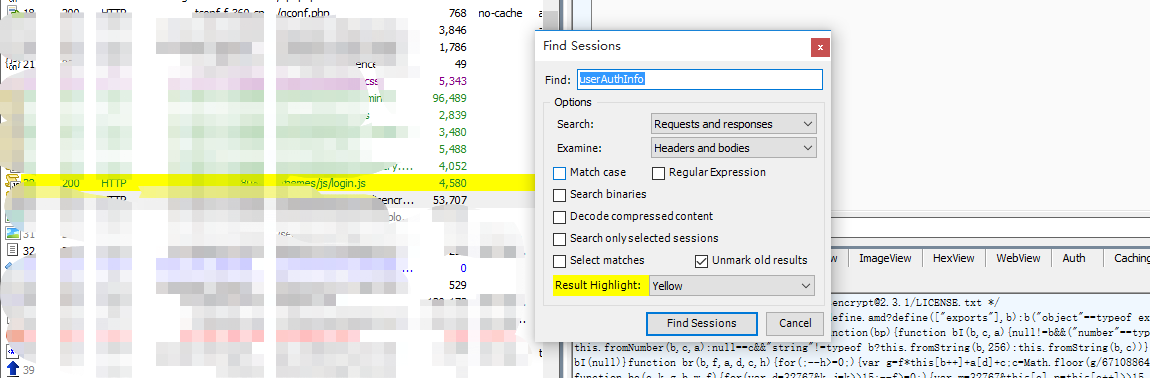python - RSA加密

RSA公开密钥密码体制。所谓的公开密钥密码体制就是使用不同的加密密钥与解密密钥，是一种“由已知加密密钥推导出解密密钥在计算上是不可行的”密码体制。

RSA算法是第一个能同时用于加密和数字签名的算法，也易于理解和操作。RSA是被研究得最广泛的公钥算法，从提出到现今的三十多年里，经历了各种攻击的考验，逐渐为人们接受，截止2017年被普遍认为是最优秀的公钥方案之一。
SET(Secure Electronic Transaction)协议中要求CA采用2048bits长的密钥，其他实体使用1024比特的密钥。RSA密钥长度随着保密级别提高，增加很快。下表列出了对同一安全级别所对应的密钥长度。$.ajax({ type: "POST", url:$("#ctx").val() + "/login.action",
data: {
userAuthInfo: getUserAuthInfo()
},
success: function (b) {
if (b.promptMsg == null) {
document.cookie = "orgTypeName =" + escape(b.orgTypeName) + ";path=/";


function getUserAuthInfo() {
var d = $("#loginName").val(); var a =$.md5($("#password").val()); var c =$("#validateCode").val();
var g = $("#loginPageType").val(); var e = d + ";" + a + ";" + c + ";" + c + ";" + g; var b =$.cookies.get("rsaPublicKey");
var f = new JSEncrypt();
f.setPublicKey(b);
return f.encrypt(e)
};


function getRSAPublicKey() {
$.ajax({ type: "POST", url:$("#ctx").val() + "/loginRSAPublicKey.action",
success: function (a) {
if (a.rsaPublicKey != null && a.rsaPublicKey != "") {
document.cookie = "rsaPublicKey =" + escape(a.rsaPublicKey) + ";path=/"
} else {
$("#validate_img").click();$("#tip").text("业务服务器异常")
}
},
error: function () {
$("#validate_img").click();$("#tip").text("业务服务器异常")
}
})
}


function getUserAuthInfo() {
var d = $("#loginName").val(); // 获取登录账号 var a =$.md5($("#password").val()); // 获取登录的密码经过md5加密 var c =$("#validateCode").val();    // 验证码的值
var g = $("#loginPageType").val(); // loginPageType同样的可通过fiddler查找找到这个值的由来。 var e = d + ";" + a + ";" + c + ";" + c + ";" + g; var b =$.cookies.get("rsaPublicKey");  // 得到加密的公钥，其中的公钥的查找可以在上一个页面上的cookies中找到，这个就简答多了。这里就不分享查找公钥的过程了。
var f = new JSEncrypt();
f.setPublicKey(b);   // rsa进行加密
return f.encrypt(e)
};


import base64
from hashlib import md5
from Crypto.Cipher import PKCS1_v1_5
from Crypto.PublicKey import RSA

e = '登录密码' + ";" + password+";" + 验证码+";"+ 验证码+";"+ "%s" % loginPageType

public_key = """-----BEGIN PUBLIC KEY-----
{rsaPublicKey}  // 加密所用到的公钥
-----END PUBLIC KEY-----""".format(rsaPublicKey=rsaPublicKey)

rsakey = RSA.importKey(public_key)
cipher = PKCS1_v1_5.new(rsakey)
cipher_text =base64.b64encode(cipher.encrypt(e))
print cipher_text